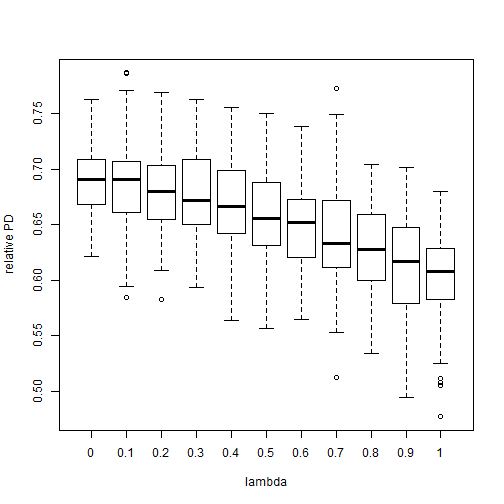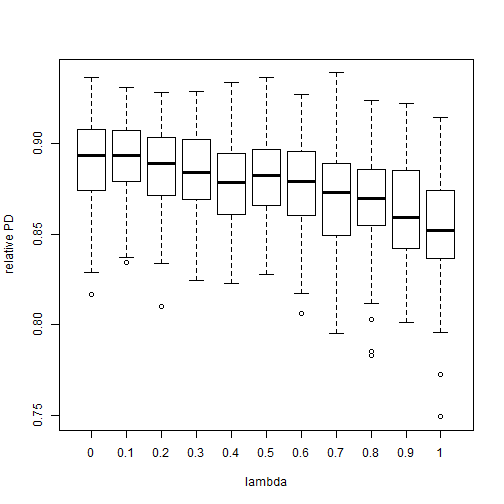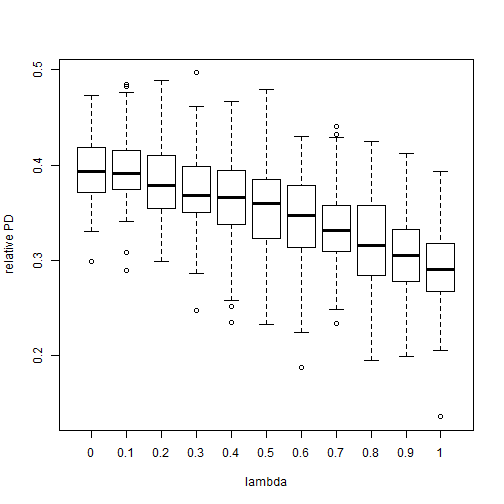## Thursday, September 18, 2014

### On the loss of phylogenetic diversity when extinction risks evolves under the threshold model

So, for a little while (though highly intermittently) I've been exploring the possibility of modeling contemporary extinction risk, specifically IUCN threat status, using the threshold model from evolutionary quantitative genetics.

In so doing, I started considering the possibility that phylogenetic signal in extinction risk could be used to make some kind of general prediction about the loss of phylogenetic diversity (PD) under conditions in which species highly vulnerable to extinction are in fact lost. Specifically in the context of the threshold model, if our process for evolution of the liability in extinction risk results in high phylogenetic signal (for instance, Brownian motion) then we would expect that the loss of PD will be high for a given extinction fraction (because whole clades will be lost). Conversely, if the process of evolution of the liability in extinction risk results in low phylogenetic signal, then we would expect the loss of PD for a given extinction fraction to be relatively low.

To illustrate this, I wrote a little bit of code that simulates extinction risk under contemporary anthropogenic conditions using the threshold model and birth-death phylogenetic simulations. We supply a phylogeny, an extinction fraction, & a particular value for λ, and then the function returns the relative PD of the tree in which vulnerable species have gone extinct compared to PD in the original tree.

``````library(phytools)
## phytools internal lambdaTree function
lambdaTree<-phytools:::lambdaTree

## custom function to simulate the loss of PD
pd.lambda<-function(tree=NULL,lambda=1,fraction=0.2){
if(is.null(tree)) tree<-pbtree(n=100,scale=1)
x<-fastBM(lambdaTree(tree,lambda))
th<-sort(x)[round(fraction*length(x))]
x<-sapply(x,threshState,thresholds=
setNames(c(th,Inf),c("extinct","extant")))
tips<-names(which(x=="extinct"))
sum(drop.tip(tree,tips)\$edge.length)/sum(tree\$edge.length)
}

## simulations for various Pagel's lambda
lambda<-0:10/10
trees<-pbtree(n=100,scale=1,nsim=100)
PD<-sapply(lambda,function(l,tt) sapply(tt,
pd.lambda,lambda=l,fraction=0.5),tt=trees)
colnames(PD)<-lambda
boxplot(PD,ylab="relative PD",xlab="lambda")
``````That's with an extinct fraction of 0.5. Let's try some other extinction fractions:

``````## extinction fraction of 0.2
PD<-sapply(lambda,function(l,tt) sapply(tt,
pd.lambda,lambda=l,fraction=0.2),tt=trees)
colnames(PD)<-lambda
boxplot(PD,ylab="relative PD",xlab="lambda")
````````````## extinction fraction of 0.8
PD<-sapply(lambda,function(l,tt) sapply(tt,
pd.lambda,lambda=l,fraction=0.8),tt=trees)
colnames(PD)<-lambda
boxplot(PD,ylab="relative PD",xlab="lambda")
``````Basically, as we predicted, when the evolutionary process for the evolution of extinction liability under contemporary circumstances results in high phylogenetic signal then loss of PD for a given extinction fraction is high. Conversely, for low phylogenetic signal loss of PD is also relatively low.

By implication, if IUCN threat status modeled on a phylogeny has high phylogenetic signal for a given clade, then the potential loss of PD given an extinction scenario in which the species identified as most vulnerable are lost will be high relative to the expected loss of PD under the same conditions if phylogenetic signal in IUCN threat status was relatively small.

That's pretty much it.